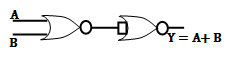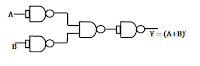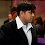## Index

 STA & SI Chapter1 Chapter2 Chapter3 Chapter4 Chapter5 Chapter6 Chapter7 Chapter8 Introduction Static Timing Analysis Clock Advance STA Signal Integrity EDA Tools Timing Models Other Topics

 Extraction & DFM Chapter1 Chapter2 Chapter3 Chapter4 Chapter5 Chapter6 Introduction Parasitic Interconnect Corner (RC Corner) Manufacturing Effects and Their Modeling Dielectric Layer Process Variation Other Topic

## Thursday, December 12, 2013

### DIGITAL BASIC - 1.3 : LOGIC GATES (Part - b)

 Index Chapter1 Chapter2 Chapter3 Chapter4 Digital Background Semiconductor Background CMOS Processing

 1.1 1.2 1.3a 1.3b 1.4 1.5 1.6 Number System Digital Arithmetic Logic Gates Logic Gates Combinational Circuits Multiplex (MUX)

In the previous post we have discussed about the basics of Logic gates (Logic levels , Different types of gates, their truth table, Boolean expression and important points/notes about gates).
As I have mentioned that NAND and NOR gates are Universal gates and we can realize any basic gates using these Universal gates. So below is the table which will help you to understand how we can use NAND/NOR gates to realize the basic gates.

Realization of Basic gates using NAND and NOR gates:

 Gate Using NAND Using NOR NOTANDORNANDNORXORXNORImportant Points to remember:
• The minimum number of NAND gates required to realize X NOR is five.
• The minimum number of NOR gates required to realize X – NOR is four.
• The minimum number of NAND gates required to realize X – OR gate is four.
• The minimum number of NOR gates required to realize X – OR gate is five.
INHIBIT CIRCUITS:
AND, OR, NAND and NOR gates can be used to control the passage of an input logic signal through to the output. When a logic signal is applied to one of the input of the logic gates, the output of the gate is decided by the other input which is known as control input B.
The logic level at this control input will determine whether the input signal is “enable to each the output” or “inhibited from reaching the output”.

A bubbled NAND gate is equivalent to OR gate.

A bubbled NOR gate is equivalent to AND gate

A bubbled AND gate is equivalent to NOR gate

A bubbled OR gate is equivalent to NAND gate

In the next section we will discuss about the Combinational and Sequential circuits in Brief which help us in VLSI later on.

1.Check out my notes also at: http://www.slideshare.net/shivoo.koteshwar/1sembasic-electronics-notesunit8digital-logic

1.nice notes sir..

2.This comment has been removed by the author.